# MP Board Class 12th Economics Important Questions Unit 3 Producer Behaviour And Supply

## MP Board Class 12th Economics Important Questions Unit 3 Producer Behaviour And Supply

### Micro Economics Producer Behaviour And Supply Important Questions

Micro Economics Producer Behaviour And Supply Objective Type Questions

Question 1.

Question 1.
There are factors of productions:
(a) Two
(b) Three
(c) Four
(d) Five
(d) Five

Question 2.
Fixed cost is also known as:
(a) Variable cost
(b) Actual cost
(c) Supplementary cost
(d) Short-term cost
(c) Supplementary cost

Question 3.
Supply falls on the same price when:
(a) Where there is decrease in supply
(b) When there is contraction in supply
(c) When supply increases
(d) When there is expansion in supply.
(a) Where there is decrease in supply

Question 4.
Active factor of production:
(a) Capital
(b) Labour
(c) Land
(d) None of these.
(b) Labour

Question 5.
In the short-run following factors are included in the process of production:
(a) Fixed factors
(b) Variable factors
(c) Both (a) and (b)
(d) None of these.
(c) Both (a) and (b)Question 2.
Fill in the blanks:

1. Short-term production function is known as ……………
2. Returns to scale is related to ……………
3. Cost incurred in per unit production is ……………
4. Increase in income from a unit of production is called ……………
5. A producer is in the state of equilibrium when he earns ……………
6. Law of supply shows …………… relation between price and supply.
7. The elasticity of supply for milk and related good is ……………

1. Law of variable proportion
2. Long term
3. Average cost
4. Marginal cost
5. Profit
6. Direct
7. Elastic.

Question 3.
State true or false:

1. Rent theory of Ricardo is based on the law of Diminishing returns.
2. Law of decreasing returns to scale arises due to Non-divisibility.
3. Fixed cost is also known as supplementary cost.
4. In case of perfect competition, a firm attains maximum satisfaction when MC curve cut MR curve.
5. There is inverse relation between price and supply.
6. The supply of perishable goods is inelastic.
7. There are four laws of production.

1. True
2. False
3. True
4. False
5. False
6. True
7. False.

Question 4.
Match the following: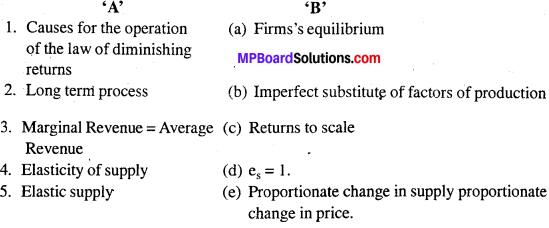1. (b)
2. (c)
3. (a)
4. (e)
5. (d).

Question 5.
Answer the following in one word/sentence:

1. Which cost is known as Transferable income?
2. Name the total of fixed cost and variable cost.
3. If the price decreases slightly, supply becomes zero, what is it known as?
4. What is addition to total revenue by the sale of an additional unit of commodity known as?
5. What profit does a firm earn in the state of equilibrium?

1. Opportunity cost
2. Total cost
3. Perfectly elastic
4. Marginal revenue
5. Maxi- mum.Producer Behaviour And Supply Very Short Answer Type Questions

Question 1.
What is production function?
Production function refers to the functional relationship between the quantity of goods produced and factors of production.

Question 2.
What is the total product of an input?
Total product means the total quantity of goods produced by a firm during a given period of time with given inputs.
TP = AP x Number of variable factor (L) or TP = $$\sum { MP }$$ – AP x Q.

Question 3.
What is average product of an input?
Average product is defined as the output produced per unit of variable input. Calculated as:
AP = TP/L or AP = TP/Q.

Question 4.
What is the marginal product of an input?
Marginal product refers to additional output produced, when one more unit of variable factor is employed.
MP = TPn – TPn -1.
MP = Change in output/Change in input
MP = ∆q / ∆ x 1.

Question 5.
What is the law of diminishing marginal product?
Law of diminishing marginal product means that when more and more units of a variable factors are employed along with a fixed factor, the marginal product of the factor must fall.

Question 6.
What is the law of variable proportion?
The law which exhibits the relationship between the units of variable factor (Keeping all other factors constant) and the amount of output in the short is known as the law of variable proportion.Question 7.
When does a production function satisfy constant returns to scale?
A production function satisfy constant returns to a scale when proportional increase in all inputs results in an increase in input by the same proportion.

Question 8.
When does a product function satisfy increasing returns to scale?
Production function satisfy increasing returns to scale when a proportional increase in all inputs result in increase in output by more than the proportions.

Question 9.
A production function satisfy decreasing return to scale when a proportional increase in all inputs result in an increase in output by less than proportions.

Question 10.
What do you mean by production cost?
Production cost refers to all sorts of monetary expenditure incurred in the production of the commodity.

Question 11.
What is fixed cost or supplementary cost?
The payment for fixed factors of production in the short-run is known as fixed cost. Fixed cost does not change with the change in the quantity of production, e.g., Rent of ‘ the factory, insurance, premium, etc.

Question 12.
What is variable cost or prime cost?
Variable cost or Prime cost:
Variable cost refers to those cost which are incurred by a firm for purchasing variables inputs like raw materials, labor fuel, electric power etc. These cost vary with the variation in the quantity produced. If the production is increased, variable cost will also increase and if the production is decreased, the variable cost will also decrease.

Question 13.
What is marginal cost?
Marginal cost is the addition to total cost caused by a small increment in output. Marginal cost may be defined as the change in total cost resulting from the unit change in the quantity produced. Thus, it can be expressed by the formula:MC=$$\frac { ∆TC}{ ∆Q }$$, ∆ = Change.

Question 14.
What is total cost?
Total cost:
The total expenditure incurred by a firm for the production of a specific quantity of a commodity is called total cost of production. The total cost increases with the increase in the quantity produced. As the production increases, total cost also increases.

Question 15.
What is opportunity cost?
Opportunity cost is defined as the value of a factor in its next best alternative use or it is the cost of forgone alternatives. It is also defined as value of next best use to which that resource could be put.

Question 16.
What is implicit cost?
Implicit costs are unrecognized costs that a firm realizes when it uses its assets and resources for one project over another. Implicit cost includes wages of the labor, rent of building, depreciation charges for machines, etc.

Question 17.
What is explicit cost?
Explicit cost refers to all those expenses made by a firm to buy goods directly. They include payments for raw material, taxes and depreciation charges, advertisement, etc.Question 18.
What do you mean by production cost ? Distinguish between the explicit cost and implicit cost
Meaning of Production cost:
Production cost refers to all sorts of monetary expenditure incurred in the production of the commodity.

Difference between Explicit cost and Implicit cost:
Explicit cost:
Cash payment made by firms to outsiders for hiring factor services and buying raw material etc. is called explicit cost.

Implicit cost:
Implicit cost self-owned and self -supplied inputs or factors of production called implicit cost.

Question 19.
What do you mean by fixed factors and variable factors of production?

1. Fixed factors:
Factors of production which do not change with the change in output e.g., land are called fixed factors.
2. Variable factors:
Variable factors of production are those factors which changes with the changes in output e.g., labor.

Producer Behavior And Supply Short Answer Type Questions

Question 1.
Write differences between Fixed Cost and Variable Cost.
Differences between Fixed cost and variable cost.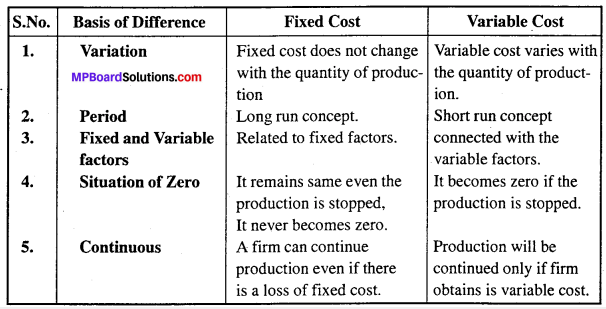Question 2.
Why cost curves are ‘U’ shaped ? Give reasons.
The important reason of beings ‘U’ shape of cost curve is, the benefits of large scale production and internal surplus of the firm. These benefits can be divided into four parts:

1. Savings in Lab-our:
Division of Lab-our and specialization is used in large scale production. It increases the efficiency and working capacity of the labor and reduces the per unit cost of production.

2. Savings in Marketing:
Selling cost is not increased in the proportion of productions. As a result per unit cost is again reduced. If a firm doubles its production then its selling expenditure like advertisement etc. will not be doubled. Hence, it will enjoy the benefit of large scale economy.

3. Managerial Savings:
Managerial expenditures decreases with the increase in the production, which is termed as managerial savings. An efficient manager, manages the business affair in the same capacity even if the volume of production increases. Hence, per unit cost is again reduced.

4. Technical Savings:
Improvement in production technology results into technical savings. Use of modern and advance technology increases production on one hand and on the other hand, it reduces per unit cost of production. The main cause of this technical saving is extension of technical cost on more units produced. Thus, from the above description it is clear that cost curves which are directly concerned with the production is shape in U letter of English alphabet.

Question 3.
Discuss the relation between Average Revenue and Marginal Revenue.
Following relationship exists between AR and MR:

1. AR increases as long as MR is higher than AR and when MR > AR, AR increases.
2. AR is maximum and constant when MR is equal to AR.
3. AR falls when MR is less than AR.
4. MP can be positive, zero and negative but AP is always positive.Question 4.
When is a consumer in a state of equilibrium?
A producer is in a state of equilibrium, if he is earning maximum profit or has profit maximization. A producer earns maximum profit where.
Profit = TR – TC, where TR>TC a producer earns abnormal profit and where TR < TC loss is also beard by the producer only.

Question 5.
What is meant by supply?
Supply refers to the quantity of a commodity which a producer or a seller or a firm is willing to sell in the market at a particular price during a given period of time.

Question 6.
What is meant by supply schedule?
Supply schedule is a tabular statement showing various quantities of a commodity being supplied at various levels of price during a given period of time. Supply schedule is of two types.

1. Individual supply schedule:
It refers to supply schedule of an individual firm in the market it shows different quantities supplied by a firm at different prices of a comm-oddities.

2. Market supply schedule:
It refers to tabular statement showing various quantities of a commodity that all the producers are willing to sell at various levels of price, during given period of time.

Question 7.
What is a supply curve?
The supply curve is a graphical representation of the relationship between the price of a good or service and the quantity supplied for a given period of time. In a typical representation the price will appear on the left vertical axis, the quantity supplied on the horizontal axis. The supply curve has two aspects:

1. Individual supply curve,
2. Market supply curve.

1. Individual supply curve:
Individual supply curve is a graphic presentation of supply schedule of an individual firm in the market.

2. Market supply curve:
Market supply curve is a graphic presentation of market supply schedule.Question 8.
Graphically explain the law of supply.
Law of supply establishes direct relationship between price and quantity of goods supplied. According to this law, supply of the commodity will increase with increase in price and decrease with decrease in price other things remaining the same. Therefore, law of supply states that keeping other determinants of supply as constant, the supply decreases with the fall in price and increase with the rise in price. It means that the price of the commodity and its supply more in the same direction.
Supply function can be written as;
S = f (P)
S = Supply of commodity
P = Price
F = Function.
The law can be explained with the help of an example: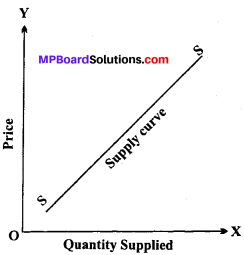The given schedule shows positive relationship between price and quantity supplied of a commodity.

Question 9.
What are the causes of movement along the supply curve and shifting of supply curve ?
When quantity supplied of a commodity changes due to change in its own price, assuming other things being equal, it is known as change in quantity supplied. When the change in supply is due to factors other than the price of the commodity, it is known as increase or decrease in supply. In order to understand the movement along the supply curve we have to understand two possibilities.

1. Increase in supply
2. Decrease in supply.

When due to changes in factors, other than price, more quantity is supplied, it is known as increase or extension in supply, inverse of this is known as decrease in supply or contraction of supply.

Question 10.
What do you understand by price elasticity of supply.
Price elasticity of supply is the responsiveness of quantity supplied when the price of the good changes. It is the ratio of the percentage change in quantity supplied to the percentage change in price.

To calculate price elasticity of supply, we use the following formula:Pes=$$\frac { ∆Q }{ ∆P }$$ x $$\frac { P }{ Q }$$

Question 11.
Explain in brief the methods of measuring price elasticity of supply.
Following methods are used to measure price elasticity of supply:

1. Percentage method,
2. Geometric method.

1. Percentage method or Proportionate method:
According to this method, price elasticity is measured as a ratio of percentage change in quantity supplied to percentage change in price of the commodity.Pes = $$\frac { \frac { \triangle Q }{ Q } x100 }{ \frac { \triangle P }{ P } x100 }$$ or Pes = $$\frac { \triangle P }{ P } x\frac { \triangle Q }{ Q }$$

2. Point method or Geometric method:
Geometric method measures elasticity at a given point on the supply curve and is also known as ‘Arc method or Point method. The following figures shows three straight line supply curves :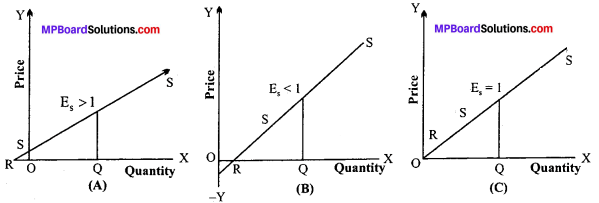Figure (A):
Supply curve is extended towards X-axis. It intersects the X-axis in its negative range at point R. The price elasticity is greater than one (RQ > OQ).

Figure (B):
The supply curve intersects the X-axis in its positive range. The price elasticity of supply is less than one as
(RQ < OQ). In the figure at point E, the elasticity of supply is less than one.

Figure (C):
The point of intersection is the origin i.e., the straight line supply curve passes through origin. The price elasticity of supply is equal to 1 (RQ = OQ).

In all three figures supply curve is a straight line. SS is the supply curve. E is the point E on the supply curve which is a straight line on the ‘X’ axis which meets at point R.
So, at point elasticity of supply, Es = $$\frac { RQ }{ OQ }$$

Where, RQ < OQ therefore, elasticity of supply at point E is less than one (Es < 1).

Question 12.
What are the factors affecting elasticity of supply?
The factors affecting elasticity of supply are:

1. Nature of the commodity
2. Cost of production
3. Time period
4. Techniques of production
5. Nature of inputs used
6. Natural constraints
7. Risk taking
8. Ability and interest of the producers.Question 13.
Write characteristic features of Average fixed cost.
Characteristic feature of average fixed cost are:

1. It slopes downward to the right from left because the total fixed cost is fixed and as the quantity of production increases, the average fixed cost decreases.
2. In the beginning, the average fixed cost slopes speedily and there after gradually.
3. It does not touch the axis and so it is in the shape of rectangular hyperbola.
4. It never becomes zero.

Question 14.
What do you mean by production cost ? Explain the difference between explicit cost and implicit cost with example.
Meaning of Production cost: The expenditure incurred by a firm on factor as well as non-factors inputs (for productions of a commodity) is called cost of production. “The cost of production of an output, then may be defined as either the purchase price or the imputed value of all productive services used in pro – ducting the output and its equivalent to the total monetary sacrifice of the firm made to secure it.

“Cost of production includes all payments made to others for goods and services as well as such items as depreciation and obsolescence. It also includes and estimated wage for services rendered by owners, plus a returns to capital and land supplied by owners.”

1. Explicit cost:
Explicit costs refer to all those expenses made by a firm to buy goods directly. They include payments for raw materials, taxes and depreciation changes, transportation, power, high fuel, advertising and so on. According to Left witch, “Explicit cost are those cash payments which firms make to outsiders for their services and goods.”

Implicit costs include three types of cost:

1. Production cost
2. Selling cost
3. Other costs.

2. Implicit cost:
Implicit costs are the imputed value of the entrepreneur’s own re-sources and services. In facts, these costs refer to the implied or unnoticed costs. They include the interest on his own capital, rent on his land, wages of his own labor etc. More – over, these costs go to the entrepreneur himself and are not recorded in practiced. In the words of Left witch implicit costs are the costs of self-owned, self-employed resources. Thus, implicit costs are unrecognized costs that a firm realizes when it uses its assets and resources for one project over another.

Implicit cost includes:

1. Salary for his own services as a manager.
2. Interest on his own capital.
3. Rent on building constructed on his own land.
4. Depreciation charges for machines etc.Question 15.
Explain the production on function of Cob-Doglus.
Production function of Cob – Doglus : Production function of Cob-Doglus is generally applicable to construction industry. According to this function quantity of production depend only on two factors labor and capital.
Formula of it is, q = $${ x }_{ 1 }^{ \alpha } , { x }_{ 2 }^{ \beta }$$
Here, α and β are positive numbers. x1 and x2 are two quantities which are used in production.

Question 16.
Explain the concept of the short run and the long run.
Short run:
Refers to a period in which output can be changed by changing only variable factors. In the short run, fixed inputs like land, building, plant machinery etc. cannot be changed. It means, production can be raised by increasing only variable factors but till the extent of fixed factors.

Long run:
Refers to a period in which output can be changed by changing all factors of production. In the long run firm can change its factory size, techniques of production, purchase new plant, machinery, patents, etc.

Question: 17.
Why AC curve is U-shaped in short run?
Or
Why AC curve U-shaped?
The shape of Average Cost (AC) depends upon Total Cost (TC). Initially, total cost (TC) increases at a diminishing rate, which makes its average i.e., Average Cost (AC) to fall,then reaches its minimum point. Thereafter, Total Cost (TC) increases at increasing rate, which makes the Average Cost (AC) to rise.This type of production behavior shows operation of law of variable proportion.In other words, the shape of the AC curve depends upon the shape of the AVC and the AFC curves. In the beginning, we find that as output increases AVC and AFC both fall, therefore, AC curve falls sharply. When AVC has started rising and AFC is falling, AC may continue to fall if the fall in AFC is more than the rise in the AVC curve.

But with further increases in output, AC would start increasing because the rise in AVC more than offsets the fall in AFC. Therefore, the shape of the AC curve would be U-shaped, first falling and than rising.

Question 18.
Explain the relationship between marginal cost and average variable cost
Relationship between Marginal cost and Average variable cost:

1. When AVC falls MC < AVC (It happens upto Q1 level of output in the diagram).
2. When AVC is minimum MC = AVC (It happens at Q1 level of output).
3. When AVC is rising MC > AVC (It happens beyond Q1 level of output).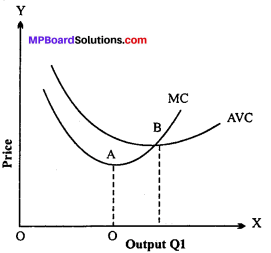Question 19.
The following table gives the total product schedule of labour. Find the corresponding average product and marginal product schedules of labour: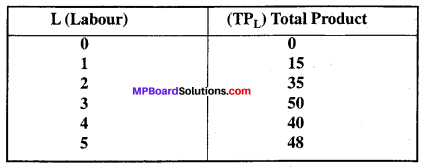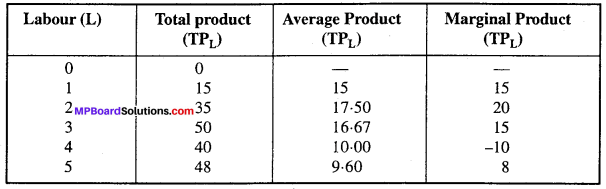Question 20.
Let the production function of a firm be q=5L$$\frac { 1 }{ 2 }$$ K $$\frac { 1 }{ 2 }$$
Find out the maximum possible output that the firm can produce with 100 units of L and 100 units of K.
Solution :
by equation q = q = 5L$$\frac { 1 }{ 2 }$$ K $$\frac { 1 }{ 2 }$$, K = 100, L = 100
q = 5(100)$$\frac { 1 }{ 2 }$$ K x (100)$$\frac { 1 }{ 2 }$$ K
q = 5$$\sqrt { 100 }$$ x V$$\sqrt { 100 }$$
q= 5 x 10 x 10
q = 500
Maximum possible out = 500 units.

Question 21.
Explain the various elements of cost of functions.
1. Meaning of Cost:
The sum of cash payment made by firms to outsiders for hiring factor services and buying raw materials etc. i.e., explicit costs and cost of self own and self supplied inputs i.e., implicit costs constitute cost of production of a commodity

2. Short run costs of production:
Short run costs are the costs during which some factors of production are in fixed supply like plant and machinery.

(a) Total Cost:
Total Cost is defined as the aggregate of all costs of production at given level of output. Total Cost (TC) is derived by the sum of Total Fixed Cost and Total Variable Cost.
TC = TFC +TVC

(b) Total Fixed Cost (TFC):
Total Fixed Costs are the sum total of expenditure incurred by the producer on the purchase or hiring of fixed factors of production. These are also called supplementary costs or overheads cost or indirect cost. The do not change with a change in output.

(c) Total Variable Cost (TVC):
Total Variable Costs are those which vary with the quantity of output produced. These are defined as the expenditure incurred by producer on the use of variable factors of production. It is very much related with the production and fluctuates with the fluctuation in production. It is also called prime cost, special costs or direct costs.

(d) Average Cost (AC):
It is the cost per unit of output produced. It is also called unit cost of production.(e) Average Fixed Cost (AFC):
It is defined as the fixed cost of producing per unit of the commodity.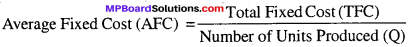(f) Average Variable Cost (AVC):
It is defined as the variable cost of producing per unit of the commodity.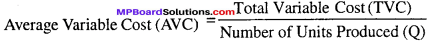(g) Marginal Cost (MC):
Marginal Cost (MC) is defined as “addition made to total variable cost or total cost when one more unit of output is produced.”
Marginal Costnth (MCn) = Total Costn – Total Costn – 1
or
Total Variable Costn -Total Variable costn – 1

Question 22.
Write main assumptions of equilibrium of the firm.
Following are the assumptions of firm’s equilibrium analysis:

1. Rational behavior:
Every firm makes an endeavor to maximize its monetary profit or its behavior is rational.
2. Minimization of cost and maximization of output:
Every firm aims at such a combination of factors of production as to attain maximum output at minimum cost.
3. Production of one commodity by one firm:
The firm’s equilibrium analysis assumes that one firm produces one commodity.
4. Perfectly elastic supply of factors of production:
The analysis takes it for granted that the supply of each factor of production is perfectly elastic. As a result, the price of each factor is constant.
5. Equal efficiency:
The analysis assumes that all the units of factors of production are equally efficient.

Producer Behavior And Supply Long Answer Type Questions

Question 1.
Explain the concepts of Average fixed costs, Average variable costs and Average total costs.
In the short period, average costs are of three types:

1. Average fixed costs
2. Average variable costs
3. Average total costs.

1. Average fixed costs:
If the total fixed costs is divided by the quantity produced, the quotient is average fixed cost. It can be expressed as:
AFC =$$\frac { TFC }{ Q}$$
Hence, AFC = Average Fixed Cost,
TFC = Total Fixed Cost,
Q = Quantity produced.

2. Average variable costs:
If we divide the total variable cost by the quantity produced, the quotient is average variable cost. It can be expressed as:
AVC = $$\frac { TVC }{ Q }$$
Here, AVC = Average Variable Cost
TVC = Total Variable Cost
Q = Quantity produced.

The average variable cost depends upon the average productivity of variable factors used in the production of a commodity. In the initial stage, the productivity increases, then remains constants and thereafter it tends to decrease. When the average productivity of variable factors increase, the average variable cost tends”to decrees and becomes constant to its minimum point. When the average productivity of variable factors starts decreasing, the average variable cost tends to increase. Thus, there is an inverse relation between productivity and cost.

3. Average Total Cost:
If we divide the total cost by the quantity produced the quotient is average total cost. It may be expressed as under:
ATC = $$\frac { TC }{ C }$$
Here, ATC = Average Total Cost,
TC = Total Cost
Q = Quantity produced.
In short period:
Total Cost = Total Fixed Cost + Total Variable Cost
ATC = $$\frac { TC}{ Q}$$
ATC = $$\frac { TFC + TVC }{ Q }$$
ATC = $$\frac { TFC }{ Q }$$ + $$\frac { TVC }{ Q }$$
ATC = AFC + AVC

Question 2.
Explain total revenue, average revenue and marginal revenue with the help of an imaginary table and diagram.
The relation of total revenue, average revenue and marginal revenue can be explained with the help of table and figure.
Table: Representation of TR, AR and MR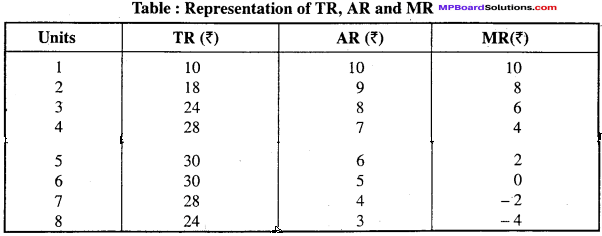The table shows that when AR is 10 TR and MR is also 10, when AR starts declining from 10 to 9 TR starts increasing from 10 to 18 and MR starts declining to 8. When AR is 8, TR is 24 and MR is 6.When AR is 5, TR is 30 and MR is 0 and after that TR starts declining and MR goes to negative. This can be explained with the help of figure.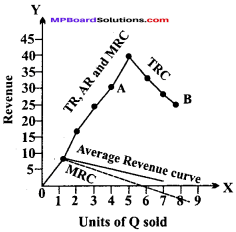1. In the figure TR is total revenue curve, AR is average revenue curve and MR is marginal revenue curve.
2. When TR started increasing AR and MR started declining. In the diagram when TR started increasing from 10 to 30 AR and MR started declining.
3. When TR is constant AR is declining but MR is ‘0’: When TR is constant that is 30 . AR start declining and MR is 0.
4. When TR started declining MR goes to negative: When TR started declining from 30 to 10, AR also declined and MR goes to negative.
5. When AR and MR both are falling, MR falls at a faster rate than AR. In other words, if AR and MR curves are downward sloping curve MR curve remains lower than
AR curve.Question 3.
Explain short period determination of price under perfect competition with example and diagram.
Prof. Marshall has given importance to time in the determination of price. According to him in price determination not only demand and supply play important role but time factor is also very important. Sometimes the demand increases suddenly but because of insufficient time the production cannot be increased according to demand. Thus, in this situation demand plays an active role and it determines the price.

Thus, on the basis of time the price can be determined in two ways:

1. Price determination under short period: Under the condition of short period, of firm cannot adjust its supply of a commodity according to the demand. Hence there may be abnormal profit or loss. Under short period the price will be determined by the equilibrium of marginal cost, average revenue and marginal revenue.

It can be explained with the help of the following diagram. Here, marginal cost and marginal revenue both meet at point ‘P‘ hence OM quantity will be sold at PM price. In other words, under the short period marginal cost, marginal revenue and average revenue are equal.

(a) In case of profit:
When demand increases the supply cannot be increased in the short run then in that time period firm earns profit. In the figure at point E, MR = MC. E is the point of maximum profit and also the point of firm equilibrium.
Total production = OQ
Price (AR) = EQ or OP
Per unit cost (AC) = RQ
Per unit profit = EQ – RQ =ER
Total profit = Per unit profit x Production = ER x OQ
= ER x PE
= MPER OQ
OQ = PE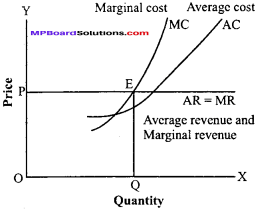(b) In case of loss:
In the short run, during production, a firm has to face loss i. e., average cost is more than average revenue. This can be cleared with the help of the diagram. In the figure MC cuts MR from below. E is the point of equilibrium where at this point OQ is the total production, EQ or OP is per unit cost, RQ is per unit cost, and per unit loss (RQ-EQ) = RE
Total = RE x MR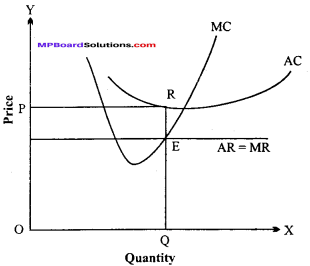Question 4.
Explain with the help of suitable example and diagram the relationship between average cost and marginal cost.
Or,
What is the relationship between average cost and marginal cost ? Explain with the help of a diagram.
This relation is exhibited by the following table and diagram:In the figure quantity produced is shown along OX axis and cost along OY axis. AC is average cost curve and MC is marginal cost curve. The shape of the curve takes U turn because of the operation of the law of decreasing returns. P is the minimum point of average cost curve. Marginal cost curve MC, cuts average cost curve AC at point P and after that point it goes on increasing. Before point P average cost curve AC and MC curve goes on decreasing. After point ‘P’ AC and MC is rising but marginal cost curve increases at an increasing- rate.Question 5.
What do you understand by production cost ? Explain marginal cost, fixed cost and variable cost.
Production is the result of effective combination of factors of production. These factors are remunerated by the firm for the contribution of their services. Payment to these factors of production forms the cost of goods. In other words, the cost refers to all expenses incurred by the firm on the production of commodity. Expenses include explicit and implicit expenses. The expenditure incurred by a firm on factor as well as non-factors inputs (for productions of a commodity) is called cost of production.

Total Cost:
The total expenditure incurred by a firm for the production of a specific quantity of a commodity is called total cost of production. The total cost increases with the increase in the quantity produced . The total cost comprises of two types of cost:

1. Fixed cost or Supplementary cost.
2. Variable cost or Prime cost.
Thus, Total Cost = Fixed cost + Variable cost.

1. Fixed cost or Supplementary cost:
It is also called general cost or indirect cost as it is not directly related with the quantity of production. It is also known as overhead cost. Thus, fixed cost is that cost which a firm incurs for the use of fixed resources like land, building, machine,organization,management, advanced technical personnel etc.
Fixed cost remains unchanged with the increase or decrease in the quantity produced. Fixed cost consists of following expenses.

1. Interest on capital
2. Rent of building
3. Depreciation on machinery
4. Salaries of permanent staff and officers etc.

2. Variable cost or Prime cost:
Variable cost refers to those cost which are incurred by a firm for purchasing variable inputs like raw materials, labor, fuel, electric power etc. This cost vary with the variation in the quantity produced. If the produced is increased, variable cost will also increase and if the production is decreased, the variable cost will also decrease. If the production is totally sloped by the firm in the short run , the variable costs will be zero. Variable costs are also called prime cost or direct cost as they are directly related with the quantity produced. In the short run, variable costs following expenditures are included

1. Price of raw material
2. Salaries of the labors
3. Fuel and electric power etc.

Thus, it is clear that Total cost is the Total of Fixed cost and Total of variable cost. The relation between average cost and marginal cost is very important for price theory. The relation between the two has the following special features.
1. Both are calculated on the basis of total cost: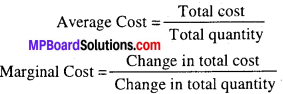2. Marginal cost curve always leads:
When average cost curve and marginal cost curve both are falling, marginal cost curve falls faster. Similarly, when average cost curve and marginal cost curve both are increasing, marginal cost curve increases faster than average cost curve. Thus, marginal cost curve always leads.

3. When average cost is minimum, the marginal cost is equal to average cost:
When average cost is lowest, the marginal cost curve cuts the average cost curve downward. In other words, here the average cost is just equal to marginal cost.

4. Both curves are of U-shape:
The averages as well as marginal cost curve are of U-shape. As long as average cost curve is falling, the marginal cost curve is below it. Similarly, when the average cost curve starts rising, the marginal cost curve is above it. If the average cost curve is U-shaped, then its corresponding marginal cost curve will cut it at its lowest point.

5. Both MC and AC are calculated from TC.Question 6.
What do you mean by equilibrium of the firm. Discuss its main features.
A firm is said to be in equilibrium when it has no incentive either to expand or to contract its output. A firm would not like to change its level of output only when it is earning maximum money profits. Hence, equilibrium is a point where firm earns maximum money profit.
Here, TR-TC is maximum.

Following are the feature of firm:

1. No change in the price of quantity of the product- In case of equilibrium, the firm does not make any change in the amount of production or value of the item. Thus, there is absence of any change.
2. Maximum profit: A firm receives maximum benefit in its equilibrium position and does not want to take any kind of risk.
3. Minimizing production cost: In its equilibrium position firm tries to minimize production cost and increase profit.
4. The firm’s equilibrium can be achieved by using total, total revenue and using marginal analysis method. There is no difference between the pricing of the product produced during equilibrium position of the firm.

Question 7.
Explain the concept of Returns to Scale. Write its three stages.
The concept of ‘Returns to scale’ studies how the output changes when all factors are changed proportionately, so that the proportion among them do not change. The definition of returns to scale is given as under, “As the quantities of inputs (factors) are varied along a particular scale line, output will vary. The responsiveness of output to such changes in output is called returns to scale.”
Returns to Scale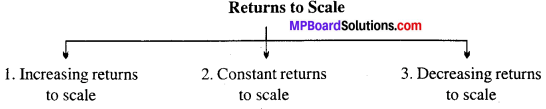1. Increasing Returns to Scale:
If the total output increases more than the proportion to the increase in inputs (factors of production), it is a case of increasing returns to scale.

For example:
if we increase all the inputs by 10 per-cent at a time, then the total output increases by more than 10 percent. Here, operates the stage of increasing returns to scale. In other words, we obtain in¬creasing returns to scale when a given percentage increase in all inputs leads to a higher percentage increase in the total output.

In the figure up to point D or equal product curve E4, the increasing returns to scale occur since BC < AB, CD < BC. It means that equal increase in output are obtained by smaller and smaller increments in inputs.2. Constant Returns to Scale:
When all the factors of production , are increased in a particular proportion, or the scale of production is increased, the quantity of production, as a result, in- u creases in the same proportion, it is a case of constant returns to scale, e.g., if we increase the quantity of all factors of production by 10 per cent and consequently the output is also increased by 10 per cent, it is called constant returns to scale.

It can diagrammatically be illustrated as the constant returns to scale with the help of equal product curve. It is assumed that in the production of goods only two factors, (labor and capital) are used.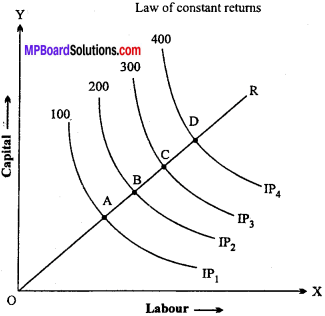In the figure, OR is the constant curve and A, B, C, D are points on the curve. These points show different quantities of labor and capital.

3. Decreasing Returns to Scale:
It occurs if a given percentage increase in all inputs results in a smaller percentage increase in output. e.g., if a car firm increases its variable inputs (capital, raw material and labor) by 50% but the output of u Lab – our – cars increases by only 35% then we say there are decreasing returns to scale from increasing the quantity of inputs.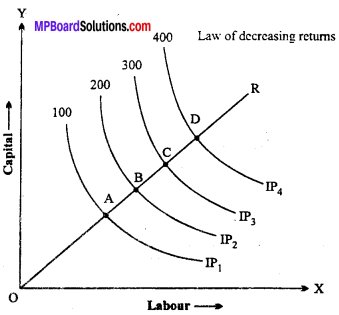Question 8.
Following table gives the schedule of Marginal product of labor, Total product of labor is zero. Find out total product and average product of labor.List of Total product and Marginal product of laborQuestion 9.
A firm’s short term marginal cost schedule is shown in the following table. The total fixed cost of firm is Rs. 100. Find out Total variable cost, Total cost, Average variable cost and Short-run average cost.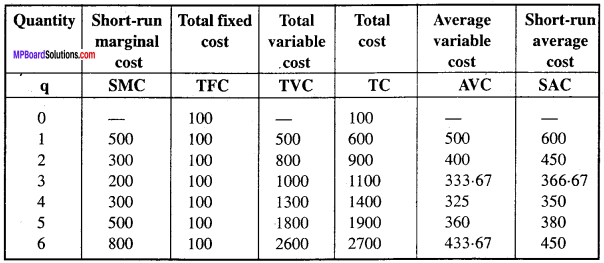Question 10.
Let the production of a firm be, Q = 2L2 K2. Find out the maximum possible output that the firm can produce with 5 unit of L and 2 units of K. What is the maxi¬mum possible output that the firm can produce with zero unit of L and 10 units of K.
Solution:
Equation, q =2 L2 K 2
Here, L= 5
K = 2
Given: q = 2 (5) 2 x (2) 2
or q = 2 (25) (4)
or q = 200 units
So, the maximum possible output will be 200 units.
In the second state:
L = 0, K = 2
q = 2 x (10) 2 x (10) 2
q = 2 x 0 x 100
q = 0
So, the maximum possible output will be zero. The maximum output would be zero with zero unit of L and 10 units of K. The reason is that the production function, our assumption is that if any input becomes zero, then the production would also be zero. Since, here labor is zero, the output would also be zero.

Question 11.
Find out the maximum possible output for a firm with zero unit of L and 10 units of K when its production function is Q = 5L + 2K.
Solution:
Equation,
q = 5L+2K L = 10,
K = 10
Given: q = 5L + 2K,
q = 5(0) + 2(10) q = 0 + 20
q = 20
So, the maximum possible output will be 20. In production function, our assumption is that if any input becomes zero, the production would also be zero. Since, here labor is 20, the output would also be 20.